Next: Example 3 Up: Examples and Troubleshooting Previous: Example 1   Contents

Example 2

The problem we want to solve is presented in section 15.1.1. We consider a system of three equations in the unknowns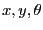: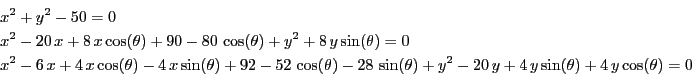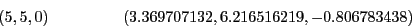By looking at the geometry of the problem it is easy to establish a rough TestDomain:

VOID SetTestDomain (INTERVAL_VECTOR & x)
{
Resize (x, 3);
x(1) = Hull (0.9,7.1);
x(2) = Hull (2.1,7.1);
x(3) = Hull (-Constant::Pi,Constant::Pi);
}
and to determine that the maximum number of real solution is 6. The IntervalFunction is written as:

INTERVAL_VECTOR IntervalTestFunction (int l1,int l2,INTERVAL_VECTOR & in)
{
INTERVAL_VECTOR xx(3);
if(l1==1)xx(1)=in(1)*in(1)+in(2)*in(2)-50.0;
if(l1<=2 && l2>=2)
xx(2)=-80.0*Cos(in(3))+90.0+(8.0*Sin(in(3))+in(2))*in(2)+(-20.0+8.0*Cos(in(3))+in(1))*in(1);
if(l2==3)
xx(3)=92.0-52.0*Cos(in(3))-28.0*Sin(in(3))+(-20.0+4.0*Sin(in(3))+
4.0*Cos(in(3))+in(2))*in(2)+(-4.0*Sin(in(3))-6.0+4.0*Cos(in(3))+in(1))*in(1);
return xx;
}
and we may use the same main program as in the previous example (the name of this program is
Test_Solve_General1).

Let's assume that we set epsilonf to 0 and epsilon to 0.01 while looking at all the solutions (Stop=0), using the maximum equation ordering and setting Dist to 0.1. The algorithm provide the following solutions,using 684 boxes: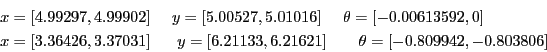We notice that indeed none of the roots are contained in the solution intervals. If we use the maximum middle-point equation ordering the algorithm provide the solution intervals, using 684 boxes: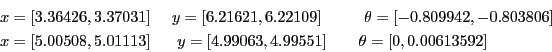which still does not contain the root (5,5,0) (but contain one of the root which show the importance of the ordering). Let's look at what is happening by setting the debug flag Debug_Level_Solve_General_Interval to 2 (see section 2.3.4.9). At some point of the process the algorithm has determined four different solution intervals: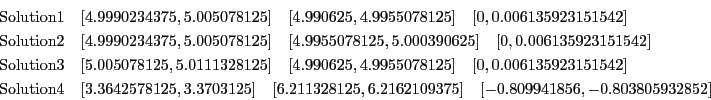the criteria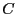for the ordering being:Clearly solution 3 has the lowest criteria and will therefore be stored as the first solution. Then solution 1 will be considered: but the distance between the middle point of solution 3 and 1 is lower than Dist and therefore solution 1 will not be retained. The solution 2 will be considered but for the same reason than for solution 1 this solution will not been retained. Finally solution 4 will be considered and it spite of his index being the worse this solution will be retained as its distance to solution 3 is greater than Dist.

Note that if the single bisection is activated and setting the flag Single_Bisection to 1 we find the two roots for epsilonf to 0 and epsilon to 0.01 with 650 boxes using the maximum equation ordering.

We may also illustrate on this example how to deal with inequalities. Assume now that we want to deal with the same system but also with the inequality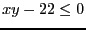. We modify the IntervalTestFunction as:

INTERVAL_VECTOR IntervalTestFunction (int l1,int l2,INTERVAL_VECTOR & in)
{
INTERVAL x,y,teta;
INTERVAL_VECTOR xx(4);

x=in(1);y=in(2);teta=in(3);
if(l1==1)xx(1)=x*x+y*y-50.0;
if(l1<=2 && l2>=2)
xx(2)=-80.0*Cos(teta)+90.0+(8.0*Sin(teta)+y)*y+(-20.0+8.0*Cos(teta)+x)*x;
if(l1<=3 && l2>=3)
xx(3)=92.0-52.0*Cos(teta)-28.0*Sin(teta)+(-20.0+4.0*Sin(teta)+
4.0*Cos(teta)+y)*y+(-4.0*Sin(teta)-6.0+4.0*Cos(teta)+x)*x;
if(l2==4)
xx(4)=x*y-22.;
return xx;
}
Part of the main program will be:

Type(1)=0;Type(2)=0;Type(3)=0;Type(4)=-1;
Num=Solve_General_Interval(3,4,Type,IntervalTestFunction,TestDomain,order,
Iterations,Stop_First_Sol,Accuracy_Variable,
Accuracy,Diff_Sol,SolutionList,6);
Here Type(4)=-1; indicates that the fourth function is an inequality of the type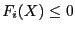. If we have to deal with the constraint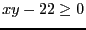then we will use Type(4)=1;.Next: Example 3 Up: Examples and Troubleshooting Previous: Example 1   Contents
Jean-Pierre Merlet 2012-12-20